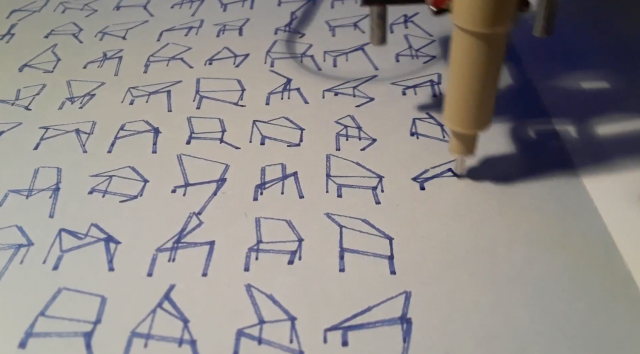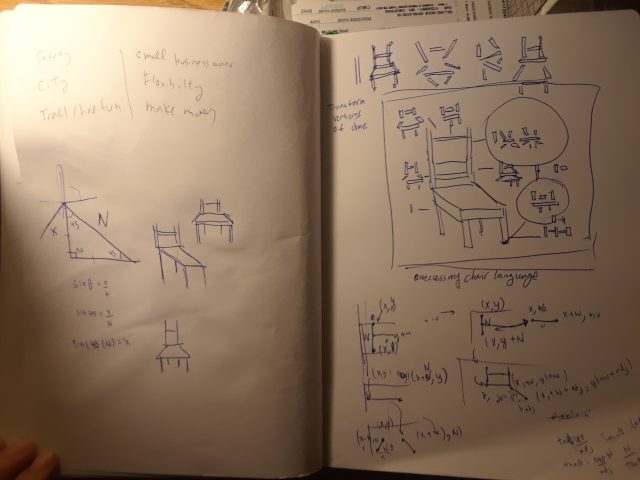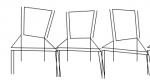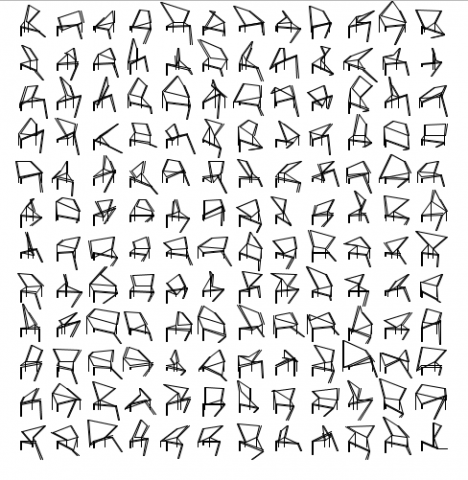# phiaq – Asemic

## AsemicI created generative chairs that are randomized in line vertices, and created a language of chairs to describe the different designs it could be based on it’s structure. At first thought of a chair language to describe a normal chair, and then it evolved into describing the different ways chairs could be constructed. When designing furniture and objects, there is always a normal set of elements that needs to be constructed. For a chair there needs to be 1) a seat 2) a place to lean your back 3) legs to hold it up. After the initial structure, furniture can be embellished to create interesting forms.

## SketchFirstly, I drew a normal chair with lines . Then, I randomized the vertices to create the messy chair language.I then created a grid with it so it looks like hieroglyphics or language. The plotting went smoothly and I did it with a light blue paper and a blue pen.Axis Draw

## ResultFor the next iteration, I want to use this language more elaborately by having it describing a chair in a diagram (kind of like the first sketch I drew) or having it in a format where it’s more purposeful in an informative design for furniture. I also should clean up my code because it’s very hard to read.

## Code

```import processing.pdf.*; boolean bRecordingPDF; int pdfOutputCount = 0;   void setup() { size(500, 500); bRecordingPDF = true; }   void keyPressed() { // When you press a key, it will initiate a PDF export bRecordingPDF = true; }   void draw() { if (bRecordingPDF) { background(255); // this should come BEFORE beginRecord() beginRecord(PDF, "myName_" + pdfOutputCount + ".pdf");   //-------------------------- // This draws a squiggly drunk walk.   noFill(); float x1 = 38; float w = 12; float y1 = 10; float w2 = w/2; float adj = w*sin(45); float adj2 = w*sin(45);//left diag float off = w/10; float lenChair = y1+w+w2+adj+off;     for (int j=0;j&lt;12;j++) { for (int f=0;f&lt;12;f++) { float aL = random(x1+(j*x1)-10,x1+(j*x1)+10); float bL = random(x1+(j*x1)-10,x1+(j*x1)+10); float aR = random(x1+w+(j*x1)-10,x1+w+(j*x1)+10); float d = random(x1+w2+adj+(j*x1)-5,x1+w2+adj+(j*x1)+5); float e = random(y1+lenChair*f-10,y1+lenChair*f+10); float g = random(x1+w2+adj+(j*x1)-10,x1+w2+adj+(j*x1)+10); float h = random(y1+w2+adj+lenChair*f-10,y1+w2+adj+lenChair*f+10); float i = random(y1+w+w2+adj2+off+lenChair*f-10,y1+w+w2+adj2+off+lenChair*f+off); float k = random(x1+w+(j*x1)-10,x1+w+(j*x1)+10); float l = random(y1+w+lenChair*f-10,y1+w+lenChair*f+10); float m = random(y1+w+w2+adj+off+lenChair*f-6,y1+w+w2+adj+off+lenChair*f+6);     line(aL,e,bL,l); //left top chair line(aL-off,e,bL-off,l); //left top chair leftest offset line(bL,l,k,y1+w+lenChair*f);//bottom connecting line line(aL,e,aR,y1+lenChair*f);//top connecting line line(aR,y1+lenChair*f,k,y1+w+lenChair*f);//right top chair line(aR+off,y1+lenChair*f,k+off,y1+w+lenChair*f);//right top chair rightest offset line(k,y1+w+lenChair*f,d,h+off);//right diag down line line(bL-off,l,x1-adj2+w2+(j*x1),y1+w2+adj2+lenChair*f);//left diag down line line(x1-adj2+w2+(j*x1),y1+w2+adj2+lenChair*f,d,h);//bottom seat line line(x1-adj2+w2+(j*x1),y1+w2+adj2+off+lenChair*f,d,h+off);//bottomest seat line off line(x1-adj2+w2+(j*x1),y1+w2+adj2+off+lenChair*f,x1-adj2+w2+(j*x1),i);//leftest bottom leg line(x1-adj2+w2+off+(j*x1),y1+w2+adj2+off+lenChair*f,x1-adj2+w2+off+(j*x1),i);//left bottom leg line(d,h+off,g,m);//right bottom leg line(d-off,h+off,g-off,m); //rightest bottom offset line(bL-off,l,bL-off,y1+w2+adj2+(w/2)+lenChair*f);//left small back leg line(k,y1+w+lenChair*f,k,y1+w2+adj+(w/2)+lenChair*f);//right small back leg   }   }     /*     line(x1,y1,x1,y1+w); //left top chair line(x1-off,y1,x1-off,y1+w); //left top chair leftest offset line(x1,y1+w,x1+w,y1+w);//bottom connecting line line(x1,w/2,x1+w,w/2);//top connecting line //rotate(random(PI,TWO_PI)); line(x1+w,y1,x1+w,y1+w);//right top chair line(x1+w+off,y1,x1+w+off,y1+w);//right top chair rightest offset line(x1+w,y1+w,x1+w2+adj,y1+w2+adj);//right diag down line line(x1,y1+w,x1-adj2+w2,y1+w2+adj2);//left diag down line line(x1-adj2+w2,y1+w2+adj2,x1+w2+adj,y1+w2+adj);//bottom seat line line(x1-adj2+w2,y1+w2+adj2+off,x1+w2+adj,y1+w2+adj+off);//bottomest seat line off line(x1-adj2+w2,y1+w2+adj2+off,x1-adj2+w2,y1+w+w2+adj2+off);//leftest bottom leg line(x1-adj2+w2+off,y1+w2+adj2+off,x1-adj2+w2+off,y1+w+w2+adj2+off); line(x1+w2+adj,y1+w2+adj+off,x1+w2+adj,y1+w+w2+adj+off);//right bottom leg line(x1+w2+adj-off,y1+w2+adj+off,x1+w2+adj-off,y1+w+w2+adj+off); line(x1,y1+w2+adj2+off,x1,y1+w2+adj2+(w/2));//left small back leg line(x1+w,y1+w2+adj+off,x1+w,y1+w2+adj+(w/2));//right small back leg */     //--------------------------   endRecord(); bRecordingPDF = false; pdfOutputCount++; } }```